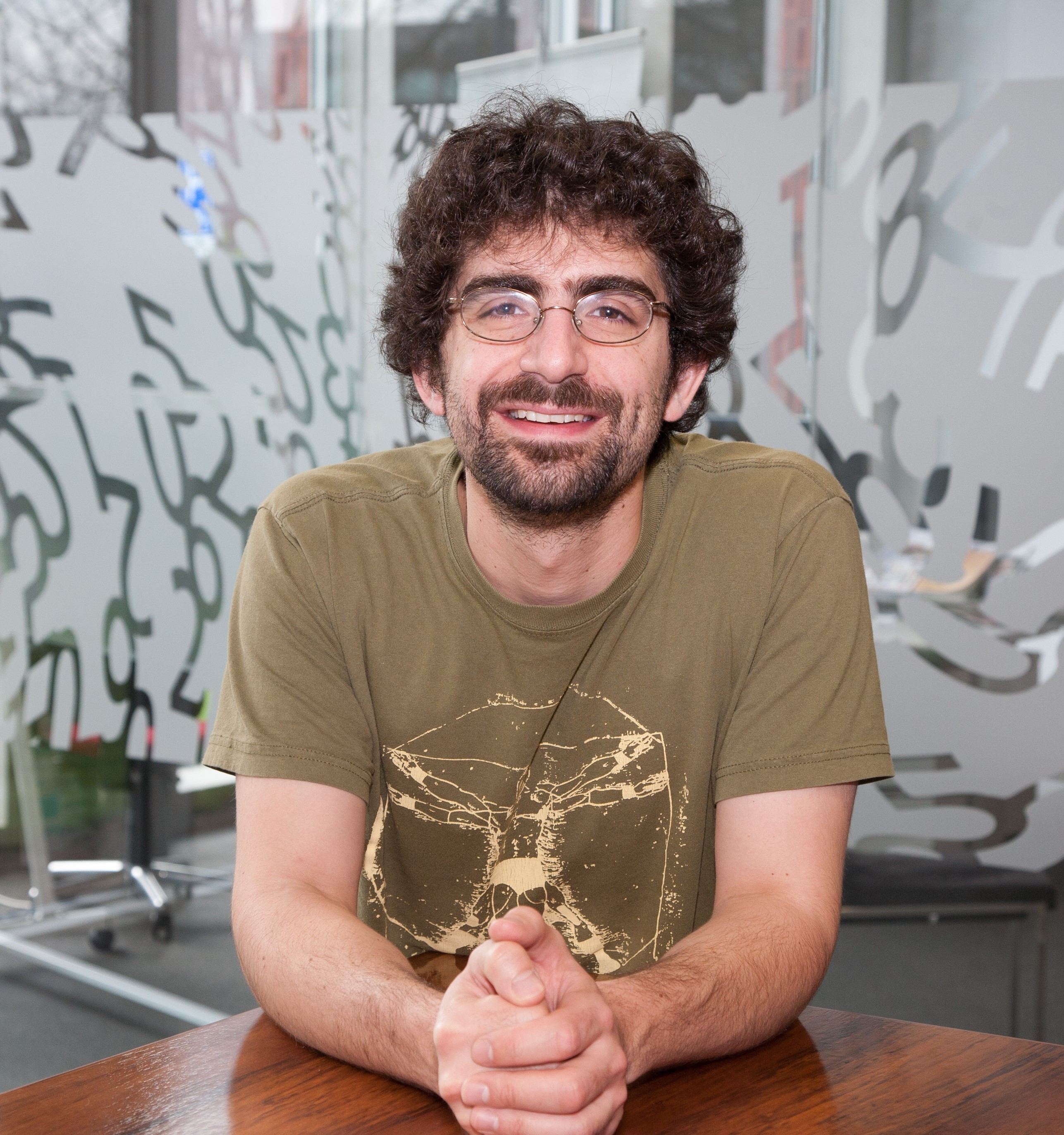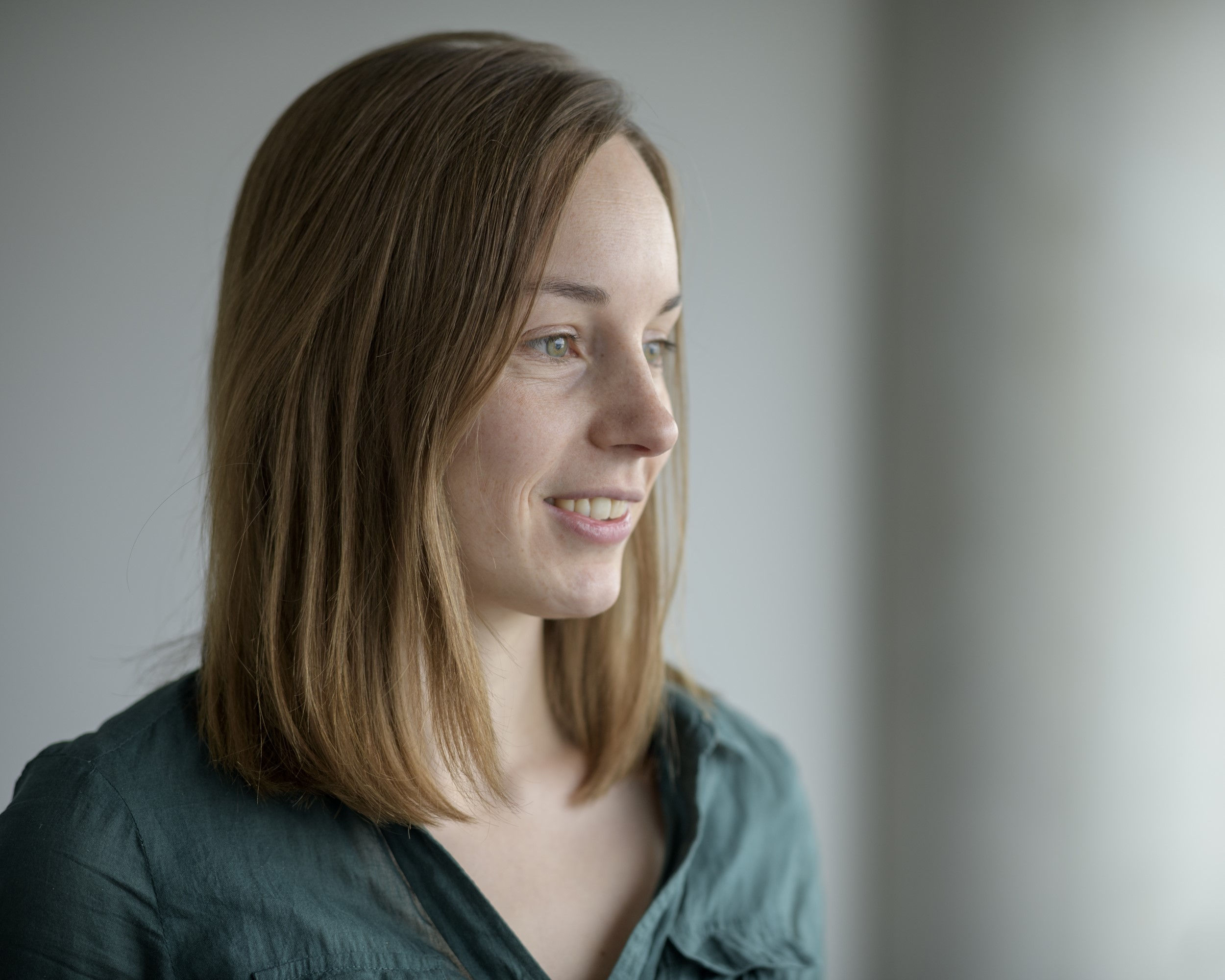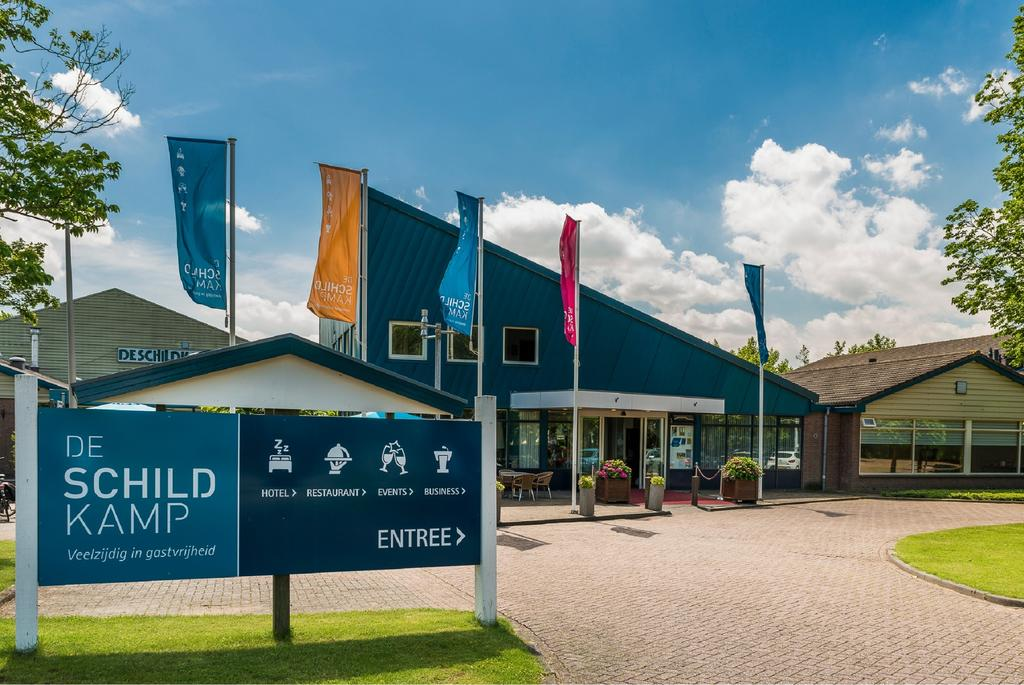# Traning week 16

On 23-27  October 2023 NETWORKS organizes the 16th Training Week in De Schildkamp in Asperen. Clara Stegehuis (UT) and Daniel Dadush (CWI) are the lecturers of the minicourses.

Next to the minicourses new NETWORKS members will introduce themselves and various Networks members will give talks about their recent results during a research presentation or open problem session. On Wednesday afternoon there will be a social event.## Circuits, Interior Point Methods and Strongly Polynomial Linear Programming

In this lecture series, I will survey recent progress on solving linear programs (LP) in strongly polynomial time, which combines tools in circuit theory from combinatorial optimization and interior point methods from continuous optimization. For a linear program in standard form min c^T x, Ax = b, x >= 0, A in R^{m x n}, a strongly polynomial solution algorithm must compute an optimal solution using a number of arithmetic operations that is polynomial in n and m (with no additional dependence on the coefficients of A,b,c). The question of whether LP can be solved in strongly polynomial time was first asked by Megiddo 83 and is listed as Smale's 9th open problem for mathematics in 21st century. Throughout the lectures, I will guide the audience through the fundamentals of circuit theory and IPMs, how to design a nearly optimal IPM from the perspective of iteration counts, and how to use circuits to upper bound the complexity of the IPM central path. This theory will allow us to recover strongly polynomial bounds for linear programs with bounded circuit imbalances (which only depend on the constraint matrix A), and to give the first strongly polynomial bound for minimum cost generalized flow, a 40 year old open problem.## Random graphs and network motifs

Mincourse by Clara Stegehuis (UT)

Network motifs, or subgraphs, can be seen as the building blocks of complex networks, and significant subgraphs often give important information on the specific network. In this minicourse, we will investigate how random graph models can help us find these significant subgraphs. We will investigate algorithmic strategies for random sampling, statistical tests and apply them on real-world network data.

## Location

Conferentiecentrum De Schildkamp, Leerdamseweg 44, 4147 BM Asperen.